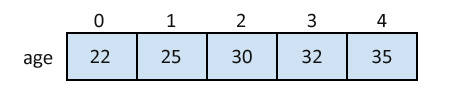# C Arrays

The array is a data structure in C programming, which can store a fixed-size sequential collection of elements of the same data type.

For example, if you want to store ten numbers, it is easier to define an array of 10 lengths, instead of defining ten variables.

In the C programming language, an array can be One-Dimensional, Two-Dimensional, and Multidimensional.

## Define an Array in C

Syntax:
``type arrayName [ size ];``

This is called a one-dimensional array. An array type can be any valid C data types, and array size must be an integer constant greater than zero.

Example:
``double amount;``

## Initialize an Array in C

Arrays can be initialized at declaration time:

``int age={22,25,30,32,35};``

Initializing each element separately in a loop:

``````int myArray;
int n = 0;

// Initializing elements of array seperately
for(n=0;n<sizeof(myArray)/sizeof(myArray);n++)
{
myArray[n] = n;
}``````

### A Pictorial Representation of the Array:## Accessing Array Elements in C

Example:
``````int myArray;
int n = 0;

// Initializing elements of array seperately
for(n=0;n<sizeof(myArray)/sizeof(myArray);n++)
{
myArray[n] = n;
}

int a = myArray; // Assigning 3rd element of array value to integer 'a'.``````